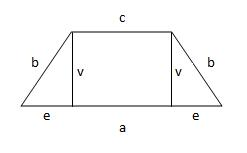# Calculated: 2839

A trapezoid is given: c = 4.2 cm, h = 8 cm, S = 42.4 cm, d = 8.4
It would help if you calculated the following: sides a and b, and perimeter o:

a =  6.4 cm
b =  8.0082 cm
o =  27.0082 cm

### Step-by-step explanation:Did you find an error or inaccuracy? Feel free to write us. Thank you!

Tips for related online calculators
The Pythagorean theorem is the base for the right triangle calculator.

#### Grade of the word problem:

We encourage you to watch this tutorial video on this math problem: